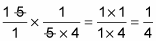##### Basic Math & Pre-Algebra All-in-One For Dummies (+ Chapter Quizzes Online)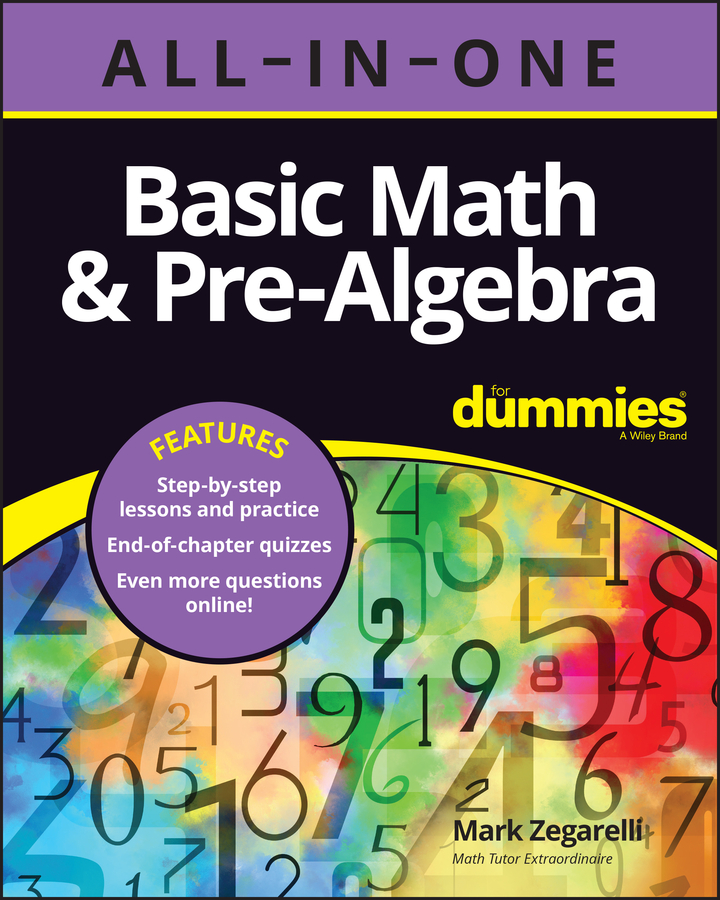To multiply two fractions, you need to have a basic knowledge of the multiplication table and the ability to reduce the answer to its lowest terms.

1. Multiply the numerators (the numbers on top) together to get the numerator of the answer.

2. Multiply the denominators (the numbers on the bottom) together to get the denominator of the answer.

For example, here’s how to multiply 2/5 by 3/7: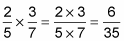Sometimes, when you multiply fractions, you may have an opportunity to reduce to lowest terms. As a rule, math people are crazy about reduced fractions, and teachers sometimes take points off a right answer if you could’ve reduced it but didn’t. Here’s a multiplication problem that ends up with an answer that’s not in its lowest terms: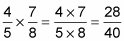Because the numerator and the denominator are both even numbers, this fraction can be reduced. Start by dividing both numbers by 2: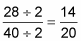Again, the numerator and the denominator are both even, so do it again: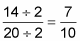This fraction is now fully reduced.

When multiplying fractions, you can often make your job easier by canceling out equal factors in the numerator and denominator. Canceling out equal factors makes the numbers that you’re multiplying smaller and easier to work with, and it also saves you the trouble of reducing at the end. Here’s how it works:

• When the numerator of one fraction and the denominator of the other are the same, change both of these numbers to 1s.

• When the numerator of one fraction and the denominator of the other are divisible by the same number, factor this number out of both. In other words, divide the numerator and denominator by that common factor.

For example, suppose you want to multiply the following two numbers: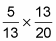You can make this problem easier by canceling out the number 13 as follows: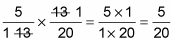You can make it even easier by noticing that 20 = 5 4, so you can factor out the number 5 as follows: